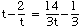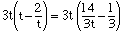Hi, my name is Lori. I'm a secondary student. How would you figure out a problem such as t-2/t =14/3t  -  1/3.   The book gave one example but it is really easy. The work YOU have to do is totally different. You don't have to tell me the answer just how to do such problems like these. thanks for listening. Hi Lori, One way to approach this problem is to find the least common multiple (lcm) of all the fractions. If you then multiply both sides of the equation by this lcm you will no longer have fractions to deal with. For your examplethe lcm of all the fractions is 3t. Thus multiply both sides by 3t to getwhich becomes 3t2 - 6t = 14 - t Now solve for t.    If you solve an equation this way then one extra step you MUST perform is to check that the answers you get are actually solutions to the equation you started with. Cheers, Penny

Go to Math Central